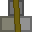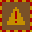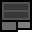# Account 347112

## BalanceMetal: 115s10 = 1850Explosives: 107s0 = 1712RC Turrets: 2

## Transfer

 PIN Receiver ReferenceMetal 16 × +Explosives 16 × +RC Turrets

## Create new account token

PIN:

New accounts cost 2 stacks (32 units) metal. You must give the token directly to the other player. Do not create the account yourself and give them the details. If you are found to do this, your account will be closed and all your items taken. You are responsible for ensuring the player you provide a new account token to knows how to use dredbank properly. If many players are found who don't know how to use dredbank properly, and they all got their accounts from one player, there may be a punishment against the player who provided their accounts.

## Transactions

Type Counterparty Account Reference NumberMetalExplosivesRC Turrets
Deposit 0 15s0 = 240 0s0 = 0 0
Sent 333000 123456 8s0 = 128 0s2 = 2 0
Sent 333000 123456 16s6 = 262 0s0 = 0 0
Sent 333000 12345 2s5 = 37 0s8 = 8 0
Deposit 0 46s0 = 736 18s0 = 288 1
Deposit 0 5s8 = 88 12s15 = 207 0
Deposit 0 12s0 = 192 5s0 = 80 0
Deposit 0 45s0 = 720 34s0 = 544 0
Deposit 0 2s0 = 32 0s0 = 0 0
Sent 483744 1324 29s0 = 464 0s0 = 0 0
Deposit 0 0s14 = 14 1s11 = 27 0
Withdrawal 0 30s0 = 480 0s0 = 0
Withdrawal 0 10s0 = 160 0s0 = 0
Deposit 0 37s0 = 592 8s0 = 128 0
Deposit 0 11s0 = 176 5s0 = 80 0
Deposit 0 15s0 = 240 7s0 = 112 0
Deposit 0 21s0 = 336 16s0 = 256 1
Sent 653090 12345 0s1 = 1 0s0 = 0 0
Deposit 0 1s0 = 16 0s0 = 0 0